#Arrange

Function sorts table rows in relation to one or several selected variables (columns).

## Properties

 Variables to Arrange Variables selected for sorting. Desc Boolean. Will be variable sorted in descending order?

## Keywords

Arrange, Sort, Reorder Rows, Arrange Table, Excel Table, Sort Rows Table, Arrange Table

## How to Sort Excel Table

Table sorting in Excel is a relatively simple task. Similarly, it is also within the Reshape.XL add-in. The advantage of the add-in solution is that you can use sorting process inside a sequence of other data processing functions. An example of the Arrange function application is the following figure.To describe the Arrange function we will use the following dataset. This dataset contains two categorical (Cat_1 and Cat_2) and three numerical variables (x, y and z).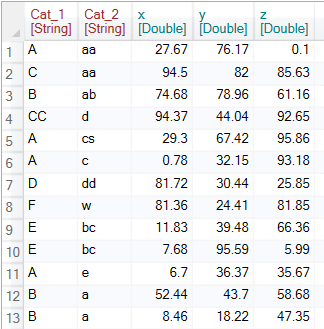To sort the dataset, click on the Arrange button in the ribbon toolbar tab Variables.The function appears in the sidebar. Clicking on it will show the setting options in the Properties Panel. In this case, it is a list of variables that you can use for sorting.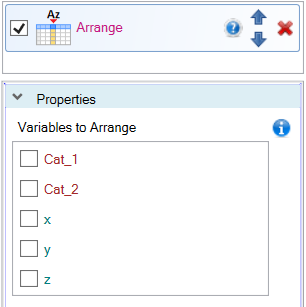This setting is very simple. Simply click the variable checkbox to sort the dataset. If the variable is selected for sorting, another checkbox is displayed - Desc, and you can define whether you want to use ascending or descending sorting. In the example (following figure), we sorted the table by the categorical variable Cat_1.The next image shows an example where we sort the dataset according to the Cat_1 variable in descending order.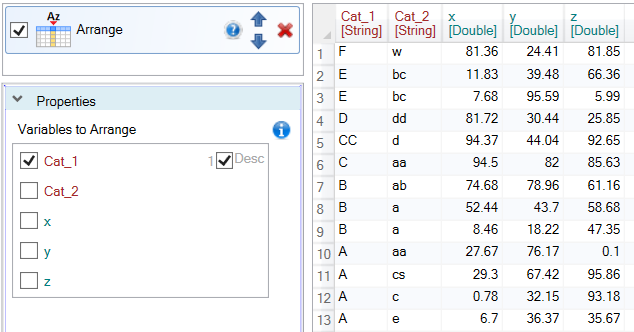The third example shows the dataset sorted by two variables - Cat_1 and Cat_2. When you use multiple variables for sorting, their order is important. This order is indicated by integer before the Desc check-box. In the example, the table is sorted in ascending order by the variable Cat_1 and subsequently by the variable Cat_2.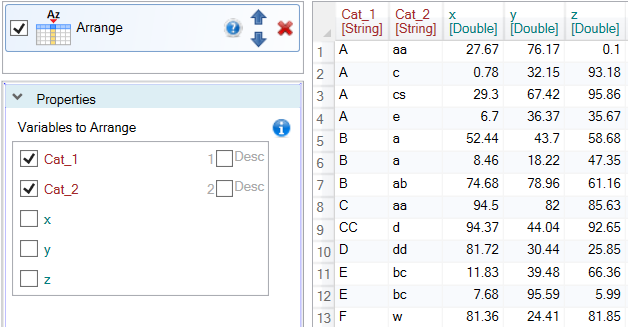The last example shows the settings in which the dataset is sorted by categorical variable Cat_1 and then by numerical variable x, from the highest values to the lowest.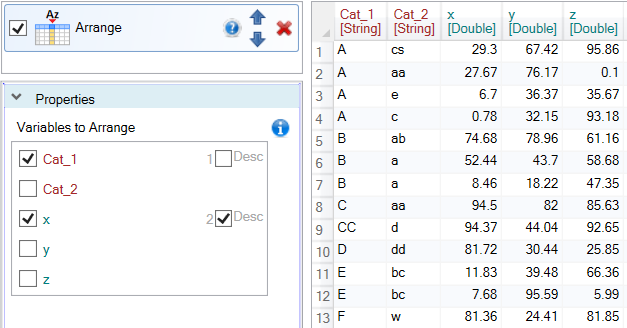Table sorting is very simple and fast - just a few clicks. The advantage of the add-in solution is that you can immediately continue to process your data using other functions. You can also deactivate or modify the sorting as needed at any time.